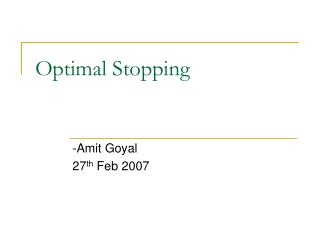DownloadDownload PresentationOptimal Stopping

# Optimal Stopping

Télécharger la présentation## Optimal Stopping

- - - - - - - - - - - - - - - - - - - - - - - - - - - E N D - - - - - - - - - - - - - - - - - - - - - - - - - - -
##### Presentation Transcript

1. Optimal Stopping -Amit Goyal 27th Feb 2007 TexPoint fonts used in EMF. Read the TexPoint manual before you delete this box.:

2. Overview • Why? • What? • How? • Example • References

3. WHY? • House selling: You have a house and wish to sell it. Each day you are offered Xn for your house, and pay k to continue advertising it. If you sell your house on day n, you will earn yn, where yn = (Xn − nk). You wish to maximise the amount you earn by choosing a stopping rule. • Secretary Problem: You are observing a sequence of objects which can be ranked from best to worst. You wish to choose a stopping rule which maximises your chance of picking the best object.

4. WHAT? (Problem Definition) • Stopping Rule is defined by two objects • A sequence of random variables, X1, X2, .., whose joint distribution is assumed known • A sequence of real-valued reward functions, y0, y1(x1), y2(x1,x2), …, y∞(x1,x2,…) • Given these objects, the problem is as follows: • You are observing the sequence of random variables, and at each step n, you can choose to either stop observing or continue • If you stop observing, you will receive the reward yn • You want to choose a stopping rule Φ to maximize your expected reward (or minimize the expected loss)

5. Stopping Rule • Stopping rule Φ consists of sequence Φ = (Φ0, Φ1(x1), Φ2(x1,x2), …) • Φn(x1,…,xn) : probability you stop after step n • 0 <= Φn(x1,…,xn) <= 1 • If N is random variable over n (time to stop), the probability mass function ψ is defined as

6. HOW?(Solution Framework) • So, the problem is to choose stopping rule Φ to maximize the expected return, V(Φ), defined as j = ∞ if we never stop (infinite horizon) j = T if we stop at T (finite horizon)

7. Optimal Stopping in Finite Horizon • Special case of general problem, by setting yT+1 = yT+2 = … y∞ = -∞ • Use DP algorithm • Here, Vj(x1,…,xj) represents the maximum return one can obtain starting from stage j having observed X1=x1,…, Xj=xj.

8. The Classical Secretary Problem (CSP) • Aka marriage problem, hiring problem, the sultan's dowry problem, the fussy suitor problem, and the best choice problem • Rules of the game: • There is a single secretarial position to fill. • There are n applicants for the position, n is known. • The applicants can be ranked from best to worst with no ties. • The applicants are interviewed sequentially in a random order, with each order being equally likely. • After each interview, the applicant is accepted or rejected. • The decision to accept or reject an applicant can be based only on the relative ranks of the applicants interviewed so far. • Rejected applicants cannot be recalled. • The object is to select the best applicant. Win: If you select the best applicant. Lose: otherwise • Note: An applicant should be accepted only if it is relatively best among those already observed

9. CSP – Solution Framework • A relatively best applicant is called a candidate • Reward Function • yj(x1,…,xn) = j/n if applicant j is a candidate, • = 0 otherwise • Lets say the interviewer rejects the first r-1 applicants and then accept the next relatively best applicant. We wish to find the optimal r

10. CSP – Solution Framework Cont. Probability that the best applicant is selected is ** (r-1)/(r-1) = 1 if r = 1

11. CSP – Solution Framework Cont. • For optimal r,

12. CSP – for large n

13. References • Optimal Stopping and Applications by Thomas S. Ferguson (http://www.math.ucla.edu/~tom/Stopping/Contents.html) • http://en.wikipedia.org/wiki/Optimal_stopping • http://en.wikipedia.org/wiki/Secretary_problem